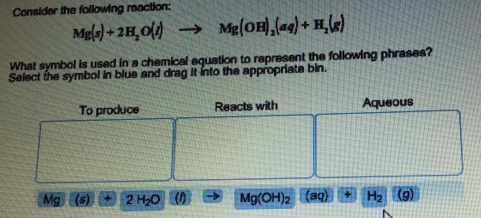# Problem: Consider the following reaction:Mg (s) + 2H2O (l) → Mg(OH)2 (aq) + H2 (g)What symbol is used in a chemical equation to represent the following phrases? Select the symbol in blue and drag in into the appropriate bin.

🤓 Based on our data, we think this question is relevant for Professor Campbell's class at WEBER.

###### FREE Expert Solution

The "To produce" group is Hγ (g)###### Problem Details

Consider the following reaction:

Mg (s) + 2H2O (l) → Mg(OH)2 (aq) + H2 (g)

What symbol is used in a chemical equation to represent the following phrases? Select the symbol in blue and drag in into the appropriate bin.